# SAT II Math II : Finding Angles with Trigonometry

## Example Questions

### Example Question #1 : Finding Angles With Trigonometry

In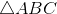: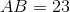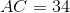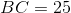Evaluate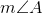to the nearest degree.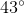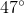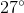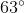Explanation:

The figure referenced is below: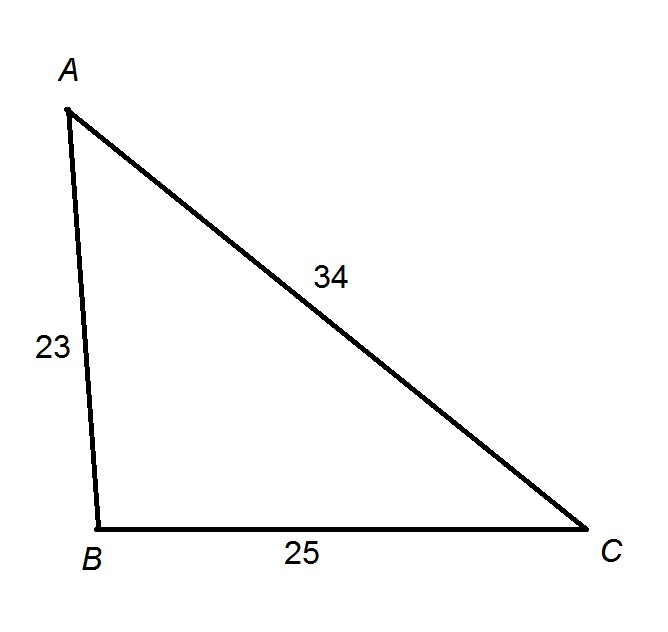By the Law of Cosines, the relationship of the measure of an angle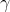of a triangle and the three side lengths, andthe sidelength opposite the aforementioned angle, is as follows: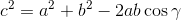All three side lengths are known, so we are solving for. Setting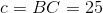, the length of the side opposite the unknown angle;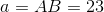;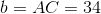;

and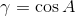,

We get the equation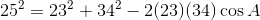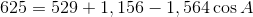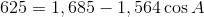Solving for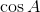: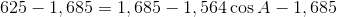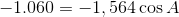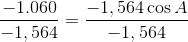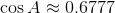Taking the inverse cosine: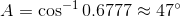,

the correct response.